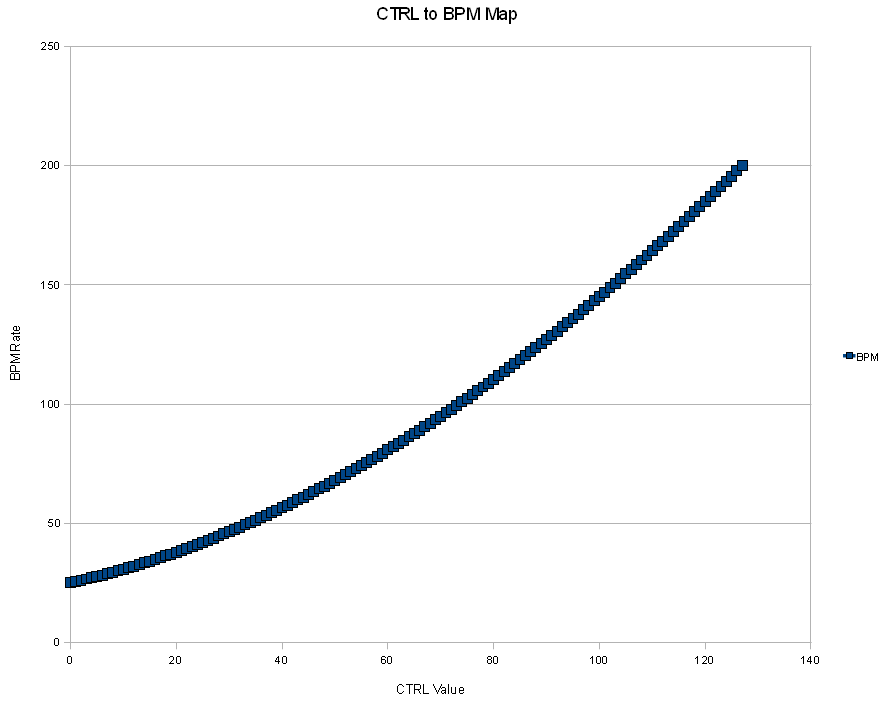Zoom G9.2tt Lago dei Cigni

## Creating a custom Controller to BPM mapping using openoffice calc or excel

December 29, 2010Comments Off on Creating a custom Controller to BPM mapping using openoffice calc or excel

Creating an input driver is easy. This article will explain how you can create custom mapping of ctrl to bpm.

Create MYINPUTDRV.INI in the subdirectory indrv.

Create this content (for example, this is for my LPD8, K1 button, use midi learn later on to override the midi input code with your own)
[DEVICECONFIGURATION]
DEVNAME=MYTESTDEVICE
[SPECIAL_COMMANDS]
INPUT0=B901E0
ACTION0=10000

[CUSTOMRANGE_TRANSFORMS]
RANGEMIN_E0=00
RANGEMAX_E0=7F
RANGEMULTIPLIER_E0=2
RANGEOFFSET_E0=0
RANGEMAP_E0=CUSTOMRANGE_MAP3

[CUSTOMRANGE_MAP3]
0=0
1=1

Then use an excel file such as those below, to create a custom range mapping.
Openoffice calc example of the CTRL to BPM mapping example
Excel version of the CTRL to BPM mapping example

Copy the values from the excel file into the INI file.
You will get something like this. (replace , by . if necessary, this depends on your regional settings).
[CUSTOMRANGE_MAP3]
0=25.158
1=25.651
2=26.16
3=26.684
4=27.224
5=27.78
6=28.351
7=28.937

This example mapping shown in a chart :Now save the INI and start midiclock, after setting the correct input code for your controller,
you can use this custom map. (and you can create any new one using calc or excel)

The resulting sample input driver is available here (rename to MYINPUTDRV.INI and place in indrv) .
enjoy !